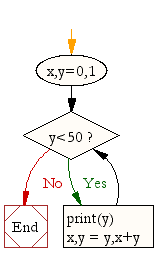## ~ OOP,Classes,Python,Tkinter ...recreating PONG

### Code and Challenge

Copy and paste the code below. Run it! Read the task and see if you can do it before moving on to the next challenge

```#note - an ERROR may be caused as Python is complaining about breaking out of the while loop when the window is closed - you can ignore that for now (or a fix is provied in the solutions section for those who are interested"""
"""
Experiment with the bouncing angle and speed
"""

from tkinter import *
import random
import time

class Ball:
def __init__(self,canvas,color):
self.canvas=canvas
self.id=canvas.create_oval(30,30,50,50,fill=color)
self.canvas.move(self.id,100,200)

#ADD THESE LINES TO OUR __INIT__ METHOD
self.x=0 #set the object variable x to 0 (don't move the ball horizontally)
self.y=-1 #set the object variable y to -1 (this means keep moving the ball UP on initilisation)
self.canvas_height=self.canvas.winfo_height() #set the canvas height by calling the canvas function winfo_height (it gives us the current canvas height)

def draw(self): #to make the ball BOUNCE we change the draw function
self.canvas.move(self.id,self.x,self.y) #change the call to the canvas's move function by passing the object variables x and y
pos=self.canvas.coords(self.id) #create a variable called pos for position - do this by calling the canvas function coordinates
#The above function would return the current x and y coordinates of anything drawn on the canvas as long as you know the identifying number
#In this case it is the oval's identifier (self.id) that gives us the coordinates
1. coords function returns the coordinates as a list of four numbers
[a,b,c,d] (e.g. 255,20,280,50)
First two numbers = top left coordinates of the oval that are x1 and y1
Last two numbers = bottom right x2 and y2 coordinates
"""

if pos <=0: #if you hit the top of the screen then stop subtracting 1 as defined in the __init__ method and therefore stop moving up -reverse directions
self.y=1
if pos >=self.canvas_height: #if the bottom coordinates are greater or equal to canvas height, then reverse again, and set y back to -1 (go up)
self.y=-1

def main():
tk=Tk()
tk.title("My 21st Century Pong Game")
tk.resizable(0,0)
tk.wm_attributes("-topmost",1)
canvas=Canvas(tk,bg="white",width=500,height=400,bd=0,highlightthickness=0)
canvas.pack()
tk.update()

ball1=Ball(canvas,'green')
while 1:
tk.update()
ball1.draw() #call the ball draw method here
time.sleep(0.01)
main()

```

Systems Life Cycle (in a nutshell): Analyse - Design - Create - Test - Evaluate. Designing something or writing out some pseudocode before you actually write code is always a good idea! Get in to the habit of doing so! You can draw your flowchart here and screenshot it.

A sample flow chart (design) for this particular challenge could look like:Each challenge section below provides an online drawing tool where you can dynamically create flowcharts. Screenshot them into your presentation for submission.